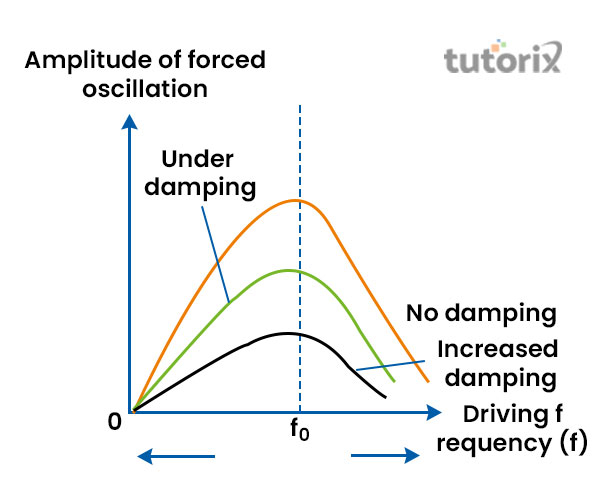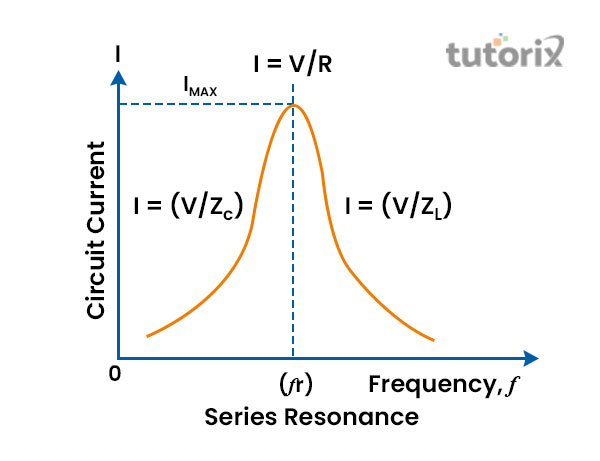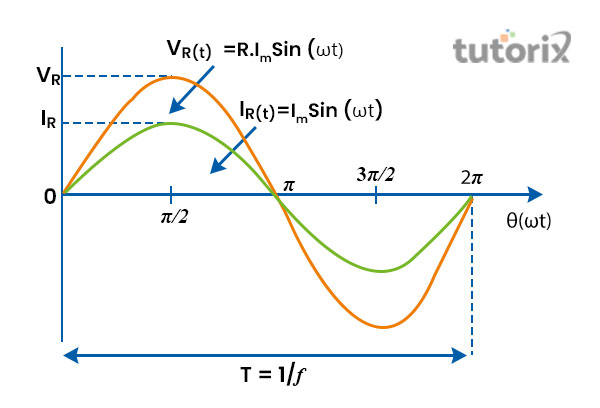# Sharpness of Resonance

## Introduction

The sharpness of resonance is clearly stated better by comprehending resonance. Resonance in physics is extremely crucial where the amplitude rises with the frequencies of excitation and the tendency of the system also rises. The sharpness of the Resonance is high when the amplitude is less and a rise in damping means maximisation of the sharpness of the resonance. In the physical world, resonance has many types including mechanical resonance, acoustic resonance and electrical resonance. The sharpness of resonance is demonstrated by the definition of resonance where the resonance tends to be sharp at the point of time. Swing is a great instance of mechanical resonance.

## What is resonance and sharpness of resonance?

Resonance is defined as the large chosen response of the system or material that vibrates in phase or step with an external applicable force of oscillation. It is illustrated as the system's tendency to swing back and forth at the highest amplitude at minimal frequencies in comparison to the others (Alwahib, Al-Rekabi & Muttlak, 2020). Resonance is known to be extremely crucial as the circuit can either dissipate or absorb the maximum energy amount at the resonance. One of the practical instances of resonance is utilised in radio receivers.Figure 1: Sharpness of resonance

"Sharpness of resonance" mainly relies on two factors including amplitude and damping. The "sharpness of resonance" is concerned with the factor of Q of the "RLC" circuit. It featured how rapidly the energy perished in the circuit of RLC that is attached to the alternating voltage (Lim et al. 2019).

(ω0/2Δω) quantity is reflected as the calculation of resonance’s sharpness. It is demonstrated from the fact that resonance is narrower or sharper if the Δω is smaller. 2Δω is demonstrated as the bandwidth and ω0 is the frequency of resonance in "Sharpness of resonance".

Amplitude in resonance's sharpness is illustrated as the wave height which is shifting in constant motion (Shahryari et al. 2021). Damping is demonstrated as the impact in which the wave’s amplitude is minimised with time.

## Illustration of Q factor

The Q factor in the sharpness of resonance is known as the quality factor which does not possess any dimensions. The q factor is utilised to feature the centre frequency and resonator’s bandwidth and the underdamped resonator. The mathematical form of the Q factor in "Sharpness of resonance" is Q = Estored/E lost per cycle. It is determined that the bandwidth of the modulated circuit minimises when the Q-factor (quality factor) maximises (Mishra, Malviya & Mishra, 2020).

Energy gets stored well in the circuit as the losses minimise so that the modulated circuit gets sharper. The Q factor relates the peak or highest energy kept in the reactance of the circuit to the dissipation or resistance of the energy during every oscillation cycle. It is referred to as the “resonant frequency” ratio to that of the bandwidth and the smaller the bandwidth means higher the circuit.

## Resonance in LCR series circuit

Resonance is mentioned as the phenomenon in the circuit when the electric circuit's output is high at a specific frequency. The frequency is determined by the inductance, conductance and resistance value in the LCR circuit (Lu et al. 2018). The series LCR circuit’s resonance happens when the capacitive and inductive reactances are similar in magnitude but cancel the other. It is 180 degrees away from the stage.Figure 2: Resonance in LCR circuit

The impedance in the LCR circuit is demonstrated by

$$\mathrm{Z=\sqrt{R^2+(X_{L}-X_{C})^2}}$$

Based on the equation, R is known to be resistance, XL is referred to as inductive resistance and XC is determined as capacitive resistance. Now, resonance in the LCR circuit is

XL = XC

$$\mathrm{\omega_{L}=\frac{1}{\omega_{C}}}$$

So, $\mathrm{\omega=\frac{1}{\sqrt{LC}}}$ which is demonstrated as the frequency of the resonance.

Moreover, impedance is minimal at the resonance, Zmin = R which reflects that current reaches the highest, Imax = Vms/R. In the LCR circuit, resonance may take place if the reactances are opposite and similar.

## Power factor at resonance

The power factor of the AC circuit and LCR circuit at the resonance is different. At the resonance, the LCR circuit acts like a pure circuit that is resistive. The impact of the inductor and capacitor cancel each other out (Li, Aphale & Zhu, 2021). LCR circuit is considered the most effective circuit for operation. It is determined that the power factor of the LCR circuit is 1.Figure 3: Power factor at resonance

The inductive reactance is similar to capacitive reactance at resonance which signifies the cancellation of voltage across capacitor and inductor. The circuit current in the above figure is in a similar phase and in the same direction (Islam et al. 2021). Hence, the phase angle between current and voltage is zero where the power factor is unity.

## Conclusion

Resonance comprises only the electrons displacement over the similar atomic nuclei which are the major feature of resonance. Glass breaking with resonant sound is one of the instances of resonance and different sorts of resonance occurring in electrical circuits is an instance of electrical resonance. It also occurs when every atom stays in a similar plane. From the overall study, it is demonstrated that amplitude is inversely proportional where damping is directly comparable to the sharpness of the resonance.

## FAQs

Q1. What is the definition of resonant frequency?

Ans: The resonant frequency is demonstrated as the object's natural frequency where it tends to vibrate at great altitude. The unit of resonant frequency is hertz. Oscillation deflection gets higher in the context of resonance.

Q2. What is defined as the Q of a coil?

Ans: Q factor or coil is referred to the unit that is dimensionless for the coil losses, a resonator or quartz. In the case of a coil, this is mentioned as the ohmic losses of the wire of the coil.

Q3. What is the connection between power and the Q factor?

Ans: Power in the "inductive and capacitive circuit" is demonstrated as Zero. Hence, the factor of circuit power is zero. However, the "Quality factor (Q)" of the circuit is the power factor’s inverse. Pure inductive and capacitive circuits are infinite (∞).

Q4. What is high bandwidth?

Ans: High bandwidth is mentioned as the frequency range within a constant frequency set which is calculated in hertz. The bandwidth of a wave is the distinction between lower and upper frequencies in a constant frequency band.

Updated on: 13-Oct-2022

822 Views